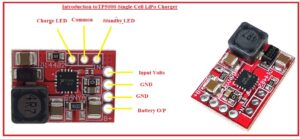Hello, readers welcome to new post. We will learn Introduction to TP5000 Single-Cell LiPo Charger.  It is the lithium-ion battery of current two amps and volts range of 4.2/3.6 can be operated. Two Amperrs is the normal category of TP500 it has a larger value of heat when high current flow but its feature is to give high speed charging.

In this post, we will discuss its pinout working, features, and some other related paramrts. So let get started.

## Introduction to TP5000 Single-Cell LiPo Charger

• This charger comprises of inductor for conversion of switches and for decoupling, current and volts adjustment, and volts sensing it consists of resistance and capacitors.
• It consists of different passive electrical components such as capacitor resistance and charger to arrange the IC as a LIPO charger.
• Its In+ pinout is used as input volts pins that have ten volts. B+ pins dispositive used for the charging of the battery.
• It has two ground pins used as reference ground. There are 2 distinct wires that can work among these pinouts to work as input and output
• There are header is configured on the board to connect LED as an indicator to show about charging and also have standby LED.### TP5000 Single-Cell LiPo Charger Features

• Some features of this module are explained here
• Its input volts are 4.5 volts to nine volts
• Its current value of two amperes.
• Its switch-mode converter gives the larger value efficiency
• The value of programmable current is 0.1 to two amperes.
• It also have constant current and constant volts modes

### TP5000 Single-Cell LiPo Charger Pinout

• Its main pinout are explained here
• IN+: It is used as input pin to give volts to board
• B+ It is the output pinout of the battery
• CHG it is charge LED pinout
• STBY: Standby led Pin
• GND it is ground